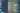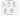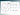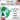April 30th, 2022

# Lesson Plan: Prime Factorization

How to teach prime factorization, math that underpins our societyEver wondered how every time you swipe your credit card, or use a messaging app to share a secret with a friend, your data stays safe? Turns out, prime factorization powers much of the cryptography that keeps the hackers away — and makes our modern society function.

I've pulled together this lesson plan to help save you time when you teach your students about prime factorization, and help make it exciting!

## First, what is prime factorization?

Prime factorization is the process breaking a number down into a set of prime numbers multiplied together.

Here's an example of prime factorization: 12 = 2 x 2 x 3.

Students may need a review of what a composite number and a prime number are before proceeding with the topic of prime factorization:

• Prime numbers are positive integers greater than 1 that are only divisible by 1 and themselves; they cannot be divided evenly by any other integer besides 1 and itself.

• Composite numbers can be divided evenly by at least one integer besides 1 or itself; for instance, 4 can be divided into smaller integers: 8 ÷ 2 = 4, so 4 is not a prime number because it can still be reduced further into smaller numbers.

If students ask, 0 and 1 are neither composite nor prime. These values will not come up when you're doing examples of finding the factors of real numbers.

## Where is prime factorization used in real life?

Students may be wondering why prime factorization is important. While it's true that the skill isn't used in every field, it serves crucial purposes in some industries:

• Finding the least common denominator. When you need to add or subtract fractions, one of the first steps is finding their least common denominator. Prime factorization can be an effective way of determining a number's prime factors (the numbers that divide it evenly).

• Solving problems in probability. To calculate probability, you often have to count how many ways a certain combination can happen using permutations and combinations. Prime factorization allows us to find out how many factors a particular number has—this can help us know how many options there are for each scenario before we begin calculating probability. For example, if we are counting the number of ways two five-card poker hands can be dealt from a standard 52-card deck with replacement, we would need to use prime factorization to determine that there are 2^5 = 32 possible outcomes for each hand. We then multiply our two sets of 32 outcomes together so see that there are 1,024 possible outcomes overall. Without knowing how many factors were involved in creating each outcome through prime factorization, we wouldn't know where to start!

• Solving problems in cryptography. Every time you pay online, cryptography prevents hackers from stealing your credit card data. Cryptography relies on the difficulty of factoring large numbers—this means that it's very unlikely someone will come up with their own encryption key based on your data unless they have access to a lot more computing power than you do. In order for them to hack your data using brute force methods by testing different keys until they find one that works, they'd need exponentially more computer time than you did when encrypting your data; this makes hacking much less likely without specialized equipment unavailable at most levels.

## How do you compute prime factorization?

Students can compute prime factorization by creating a factor tree. For students that need a visual walk through, I find this Khan Academy video helpful:

Here's the steps to create a factor tree:

1. First, you'll want to start by writing down your number (the number you want to find the prime factors of) in the center circle and drawing two branches coming off of it.

2. Then divide your number by one of its factors that you think may be prime—I usually just start with 2 and work my way up—and write down those two numbers at the ends of each branch.

3. Repeat the process with those numbers until either both endpoints are prime or they break down into another set of numbers whose product equals the original number.

I always circle the prime number at the endpoint so that it's clear when I've stopped.

Here's some examples that you can walk through with your students:### Prime factorization of 24

24 = 2 x 2 x 2 x 3

### Prime factorization of 36

36 = 2 x 2 x 3 x 3

### Prime factorization of 48

48 = 2 x 2 x 2 x 2 x 3

### Prime factorization of 72

72 = 2 x 2 x 2 x 3 x 3

### Prime factorization of 100

100 = 2 x 2 x 5 x 5

## What's a fun way to practice prime factorization?

I've created a print-and-go activity for you called Prime Factorization Doodle Math.

It includes three levels that help students practice finding the factors of 2-, 3- and 4-digit numbers. Each answer unlocks patterns that they can doodle onto an image. At the end of class, each student leaves with a masterpiece (or two or three)!

Here is a sneek peek of the activity!Give it a try in your classroom:## Want more ideas and freebies?

We'll send you 6 activities (66 pages!) — for free.

Engaging3rd - 7th GradeLow-or-No-Prep

#### Seasons

Made with ❤️ in sunny California 🌴